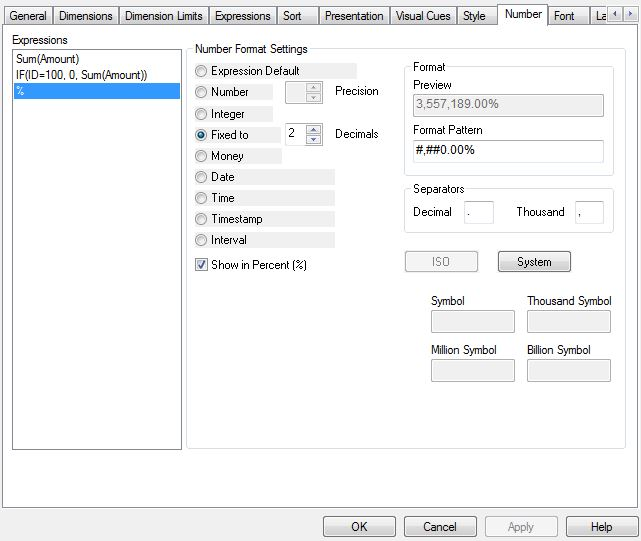# New to QlikView

Discussion board where members can get started with QlikView.

New Contributor II

## Issue with multiple number formats in a pivot table

I have an unusual issue with number formatting in a pivot table I have created.  Basically I need to be able to show some measures as percentages and some as whole numbers.  To do this I have specified the number formats in the expression as follows:

=if(match([Measure ID],11,226,227,228) num(sum(Measures.Numerator),'#,##0'),

num(Measures.Numerator/Measures.Denominator, '#.#0%'))

Where this doesn't work is if the whole number is a 1 then this displays as 100.00% and conversely if the percentage is 0 then I want it to show as 0.00% but it's showing as 0Has anyone got a workaround for this?

Thanks

1 Solution

Accepted Solutions
New Contributor II

## Re: Issue with multiple number formats in a pivot table

None of the straight forward number formatting changes worked, so have found a workaround whereby in the load if the value is zero I change this to 0.00000001 so it forces the percentage calculation but has no effect on how the 0.00% is shown in the table.

Thanks all for your help though4 RepliesMVP

## Re: Issue with multiple number formats in a pivot table

Would you be able to share you file to take a look at this?

Valued Contributor II

## Re: Issue with multiple number formats in a pivot table

Goto Number tab ==> Set Number Format Settings as belowValued Contributor II

## Re: Issue with multiple number formats in a pivot table

Use this format...

This is working for me....Zero's as well showing 0.00%.

=  #,##0.00%

IF(ID=100, Num(Sum(Amount)/Sum(TOTAL Amount),'#,##0.00%'), Num(Sum(Amount),'#,##0' ))

New Contributor II

## Re: Issue with multiple number formats in a pivot table

None of the straight forward number formatting changes worked, so have found a workaround whereby in the load if the value is zero I change this to 0.00000001 so it forces the percentage calculation but has no effect on how the 0.00% is shown in the table.

Thanks all for your help though